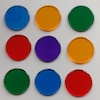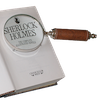# Resources tagged with: Factors and multiples

Filter by: Content type:
Age range:
Challenge level:

### There are 156 results

Broad Topics > Properties of Numbers > Factors and multiples### Scoring with Dice

##### Age 7 to 11Challenge Level

I throw three dice and get 5, 3 and 2. Add the scores on the three dice. What do you get? Now multiply the scores. What do you notice?### Divide it Out

##### Age 7 to 11Challenge Level

What is the lowest number which always leaves a remainder of 1 when divided by each of the numbers from 2 to 10?### A Mixed-up Clock

##### Age 7 to 11Challenge Level

There is a clock-face where the numbers have become all mixed up. Can you find out where all the numbers have got to from these ten statements?### Odds and Threes

##### Age 7 to 11Challenge Level

A game for 2 people using a pack of cards Turn over 2 cards and try to make an odd number or a multiple of 3.### Multiplication Squares

##### Age 7 to 11Challenge Level

Can you work out the arrangement of the digits in the square so that the given products are correct? The numbers 1 - 9 may be used once and once only.### Abundant Numbers

##### Age 7 to 11Challenge Level

48 is called an abundant number because it is less than the sum of its factors (without itself). Can you find some more abundant numbers?### Ducking and Dividing

##### Age 7 to 11Challenge Level

Your vessel, the Starship Diophantus, has become damaged in deep space. Can you use your knowledge of times tables and some lightning reflexes to survive?### The Moons of Vuvv

##### Age 7 to 11Challenge Level

The planet of Vuvv has seven moons. Can you work out how long it is between each super-eclipse?### Dicey Array

##### Age 5 to 11Challenge Level

Watch the video of this game being played. Can you work out the rules? Which dice totals are good to get, and why?### Number Tracks

##### Age 7 to 11Challenge Level

Benâ€™s class were cutting up number tracks. First they cut them into twos and added up the numbers on each piece. What patterns could they see?### What Is Ziffle?

##### Age 7 to 11Challenge Level

Can you work out what a ziffle is on the planet Zargon?### Zios and Zepts

##### Age 7 to 11Challenge Level

On the planet Vuv there are two sorts of creatures. The Zios have 3 legs and the Zepts have 7 legs. The great planetary explorer Nico counted 52 legs. How many Zios and how many Zepts were there?### Mystery Matrix

##### Age 7 to 11Challenge Level

Can you fill in this table square? The numbers 2 -12 were used to generate it with just one number used twice.### Making Pathways

##### Age 7 to 11Challenge Level

Can you find different ways of creating paths using these paving slabs?### Being Resilient - Primary Number

##### Age 5 to 11Challenge Level

Number problems at primary level that may require resilience.### Being Collaborative - Primary Number

##### Age 5 to 11Challenge Level

Number problems at primary level to work on with others.### What Two ...?

##### Age 7 to 11 ShortChallenge Level

56 406 is the product of two consecutive numbers. What are these two numbers?### Which Is Quicker?

##### Age 7 to 11Challenge Level

Which is quicker, counting up to 30 in ones or counting up to 300 in tens? Why?### Fitted

##### Age 7 to 11Challenge Level

Nine squares with side lengths 1, 4, 7, 8, 9, 10, 14, 15, and 18 cm can be fitted together to form a rectangle. What are the dimensions of the rectangle?### It Figures

##### Age 7 to 11Challenge Level

Suppose we allow ourselves to use three numbers less than 10 and multiply them together. How many different products can you find? How do you know you've got them all?### Two Primes Make One Square

##### Age 7 to 11Challenge Level

Can you make square numbers by adding two prime numbers together?### A First Product Sudoku

##### Age 7 to 14Challenge Level

Given the products of adjacent cells, can you complete this Sudoku?### American Billions

##### Age 11 to 14Challenge Level

Play the divisibility game to create numbers in which the first two digits make a number divisible by 2, the first three digits make a number divisible by 3...### Multiply Multiples 1

##### Age 7 to 11Challenge Level

Can you complete this calculation by filling in the missing numbers? In how many different ways can you do it?### Multiply Multiples 2

##### Age 7 to 11Challenge Level

Can you work out some different ways to balance this equation?### Multiply Multiples 3

##### Age 7 to 11Challenge Level

Have a go at balancing this equation. Can you find different ways of doing it?### Fractions in a Box

##### Age 7 to 11Challenge Level

The discs for this game are kept in a flat square box with a square hole for each. Use the information to find out how many discs of each colour there are in the box.### Multiples Sudoku

##### Age 11 to 14Challenge Level

Each clue in this Sudoku is the product of the two numbers in adjacent cells.### Tom's Number

##### Age 7 to 11Challenge Level

Work out Tom's number from the answers he gives his friend. He will only answer 'yes' or 'no'.### The Remainders Game

##### Age 7 to 14Challenge Level

Play this game and see if you can figure out the computer's chosen number.### Product Sudoku

##### Age 11 to 16Challenge Level

The clues for this Sudoku are the product of the numbers in adjacent squares.### Number Detective

##### Age 5 to 11Challenge Level

Follow the clues to find the mystery number.### Times Right

##### Age 11 to 16Challenge Level

Using the digits 1, 2, 3, 4, 5, 6, 7 and 8, mulitply a two two digit numbers are multiplied to give a four digit number, so that the expression is correct. How many different solutions can you find?### Just Repeat

##### Age 11 to 14Challenge Level

Think of any three-digit number. Repeat the digits. The 6-digit number that you end up with is divisible by 91. Is this a coincidence?### Factor-multiple Chains

##### Age 7 to 11Challenge Level

Can you see how these factor-multiple chains work? Find the chain which contains the smallest possible numbers. How about the largest possible numbers?### Seven Flipped

##### Age 7 to 11Challenge Level

Investigate the smallest number of moves it takes to turn these mats upside-down if you can only turn exactly three at a time.### Diagonal Product Sudoku

##### Age 11 to 16Challenge Level

Given the products of diagonally opposite cells - can you complete this Sudoku?### Gran, How Old Are You?

##### Age 7 to 11Challenge Level

When Charlie asked his grandmother how old she is, he didn't get a straightforward reply! Can you work out how old she is?### What Do You Need?

##### Age 7 to 11Challenge Level

Four of these clues are needed to find the chosen number on this grid and four are true but do nothing to help in finding the number. Can you sort out the clues and find the number?##### Age 11 to 16Challenge Level

The items in the shopping basket add and multiply to give the same amount. What could their prices be?### Big Powers

##### Age 11 to 16Challenge Level

Three people chose this as a favourite problem. It is the sort of problem that needs thinking time - but once the connection is made it gives access to many similar ideas.### Eminit

##### Age 11 to 14Challenge Level

The number 8888...88M9999...99 is divisible by 7 and it starts with the digit 8 repeated 50 times and ends with the digit 9 repeated 50 times. What is the value of the digit M?### Thirty Six Exactly

##### Age 11 to 14Challenge Level

The number 12 = 2^2 × 3 has 6 factors. What is the smallest natural number with exactly 36 factors?### Table Patterns Go Wild!

##### Age 7 to 11Challenge Level

Nearly all of us have made table patterns on hundred squares, that is 10 by 10 grids. This problem looks at the patterns on differently sized square grids.### Down to Nothing

##### Age 7 to 11Challenge Level

A game for 2 or more people. Starting with 100, subratct a number from 1 to 9 from the total. You score for making an odd number, a number ending in 0 or a multiple of 6.### Times Tables Shifts

##### Age 7 to 11Challenge Level

In this activity, the computer chooses a times table and shifts it. Can you work out the table and the shift each time?### Neighbours

##### Age 7 to 11Challenge Level

In a square in which the houses are evenly spaced, numbers 3 and 10 are opposite each other. What is the smallest and what is the largest possible number of houses in the square?### Money Measure

##### Age 7 to 11Challenge Level

How can you use just one weighing to find out which box contains the lighter ten coins out of the ten boxes?### Factoring Factorials

##### Age 11 to 14Challenge Level

Find the highest power of 11 that will divide into 1000! exactly.### Multiplication Series: Number Arrays

##### Age 5 to 11

This article for teachers describes how number arrays can be a useful representation for many number concepts.